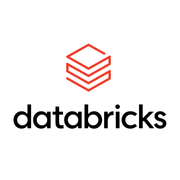## 이 전문 분야 정보

최근 조회 1,517
The purpose of this series of courses is to teach the basics of Computational Statistics for the purpose of performing inference to aspiring or new Data Scientists. This is not intended to be a comprehensive course that teaches the basics of statistics and probability nor does it cover Frequentist statistical techniques based on the Null Hypothesis Significance Testing (NHST). What it does cover is: The basics of Bayesian statistics and probability Understanding Bayesian inference and how it works The bare-minimum set of tools and a body of knowledge required to perform Bayesian inference in Python, i.e. the PyData stack of NumPy, Pandas, Scipy, Matplotlib, Seaborn and Plot.ly A scalable Python-based framework for performing Bayesian inference, i.e. PyMC3 With this goal in mind, the content is divided into the following three main sections (courses). Introduction to Bayesian Statistics - The attendees will start off by learning the the basics of probability, Bayesian modeling and inference in Course 1. Introduction to Monte Carlo Methods - This will be followed by a series of lectures on how to perform inference approximately when exact calculations are not viable in Course 2. PyMC3 for Bayesian Modeling and Inference - PyMC3 will be introduced along with its application to some real world scenarios. The lectures will be delivered through Jupyter notebooks and the attendees are expected to interact with the notebooks.
공유 가능한 수료증
완료 시 수료증 획득
100% 온라인 강좌
지금 바로 시작해 나만의 일정에 따라 학습을 진행하세요.
유연한 일정
유연한 마감을 설정하고 유지 관리합니다.
초급 단계
완료하는 데 약 3개월이 걸립니다
권장 학습 속도: 주 4시간
영어
공유 가능한 수료증
완료 시 수료증 획득
100% 온라인 강좌
지금 바로 시작해 나만의 일정에 따라 학습을 진행하세요.
유연한 일정
유연한 마감을 설정하고 유지 관리합니다.
초급 단계
완료하는 데 약 3개월이 걸립니다
권장 학습 속도: 주 4시간
영어

## 특화 과정 이용 방법

### 강좌 수강

Coursera 특화 과정은 한 가지 기술을 완벽하게 습득하는 데 도움이 되는 일련의 강좌입니다. 시작하려면 특화 과정에 직접 등록하거나 강좌를 둘러보고 원하는 강좌를 선택하세요. 특화 과정에 속하는 강좌에 등록하면 해당 특화 과정 전체에 자동으로 등록됩니다. 단 하나의 강좌만 수료할 수도 있으며, 학습을 일시 중지하거나 언제든 구독을 종료할 수 있습니다. 학습자 대시보드를 방문하여 강좌 등록 상태와 진도를 추적해 보세요.

### 실습 프로젝트

모든 특화 과정에는 실습 프로젝트가 포함되어 있습니다. 특화 과정을 완료하고 수료증을 받으려면 프로젝트를 성공적으로 마쳐야 합니다. 특화 과정에 별도의 실습 프로젝트 강좌가 포함되어 있는 경우, 다른 모든 강좌를 완료해야 프로젝트 강좌를 시작할 수 있습니다.

### 수료증 취득

모든 강좌를 마치고 실습 프로젝트를 완료하면 취업할 때나 전문가 네트워크에 진입할 때 제시할 수 있는 수료증을 취득할 수 있습니다.강좌1

3.2
별점
22개의 평가
11개의 리뷰
강좌2

3.0
별점
10개의 평가
2개의 리뷰
강좌3

3.5
별점
10개의 평가
5개의 리뷰

## 제공자:## 자주 묻는 질문

궁금한 점이 더 있으신가요? 학습자 도움말 센터를 방문해 보세요.